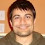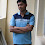# Bubble sort in Java - Program to sort an Integer Array [Example]

Bubble sort is one of the classic sorting algorithms,s which is used to explain sorting during various computer and engineering courses. Because of its algorithmic nature and simplicity, it's often used in various Java and C++ programming exercises. You may expect questions like the Write Java program to sort integer arrays using bubble sort during any programming interview. Since algorithmic questions are always tricky questions and not easy to code. Even the simplest of them can lead to confusion, especially if you are not gifted with a natural programming head. I have seen many developers fumble if asked to code on the spot. That's why it's advisable to do algorithmic and logical programming during training and learning programming and OOPS to get this skill of converting logic into code.

Let's come back to Bubble sort, In the Bubble sort algorithm we sort an unsorted array by starting from the first element and comparing it with the adjacent element. If the former is greater than later then we swap and by doing this we get the largest number at the end after the first iteration.

So in order to sort n elements you require n-1 iteration and almost n-1 comparison. To recap here is the logic for the bubble sort sorting algorithm :

1) Start comparing a to a

2) If a > a then swap numbers e.g. a=a and a=a

3) compare a to a and repeat till you compare last pair

4) This is referred to as one pass and at the end of the first pass largest, the number is at last position and already sorted.

5) Just repeat this comparison again starting from a but this time going till second last pair only

## How to sort integer array using bubble sort in Java

Here is a complete code example of a bubble sort in Java. It uses the same algorithm as explained in the first pass, it uses two loops. The inner loop is used to compare adjacent elements and the outer loop is used to perform Iteration. because of using two loops, it results in an order of n^2 which is not great in terms of performance.

If you are using Array List instead of the array then you can sort them using Collections.sort method for better performance, for details, check How to sort Array List in ascending and descending order.

Bubble sort is a good sorting algorithm to start with but you also need to learn more difficult and useful ones like QuickSortMergeSort, HeapSort, as well as some advanced constant time, also known as O(n) sorting algorithms like Radix SortBucket Sort, and Counting Sort if you want to do well on technical interviews.

If you are preparing for coding interviews then I suggest you double down into Algorithms as it takes time to develop the coding sense. I also recommend you check out Grokking the Coding Interview: Patterns for Coding Questions on Educative, an interactive portal for coding interviews to learn some useful patterns which can help you to solve many common coding problems.

Anyway, Now, let's see the Java program which implements this bubble sort logic to sort unsorted integer arrays.

package test;

import java.util.Arrays;

/**
* Java program to sort integer array using bubble sort sorting algorithm.
* bubble sort is one of the simplest sorting algorithm but performance
* of bubble sort is not good, it's average and worst-case performance
* ranges in O(n2) and that's why it is not used to sort a large set of
* unsorted data. Bubble sort can be used for educational and testing
* purpose to sort a small number of data to avoid the performance penalty.
* This program is also a good example of how to print contents of Array in Java
*
* @author http://java67.blogspot.com
*/

public class BubbleSort {

public static void main(String args[]) {
//testing our bubble sort method in Java
int[] unsorted = {32, 39,21, 45, 23, 3};
bubbleSort(unsorted);

//one more testing of our bubble sort code logic in Java
int[] test = { 5, 3, 2, 1};
bubbleSort(test);

}

/*
* In bubble sort we need n-1 iteration to sort n elements
* at end of first iteration larget number is sorted and subsequently numbers smaller
* than that.
*/

public static void bubbleSort(int[] unsorted){
System.out.println("unsorted array before sorting : " + Arrays.toString(unsorted));

// Outer loop - need n-1 iteration to sort n elements
for(int i=0; i<unsorted.length -1; i++){

//Inner loop to perform the comparison and swapping between adjacent numbers
//After each iteration one index from last is sorted
for(int j= 1; j<unsorted.length -i; j++){

//If the current number is greater than swap those two
if(unsorted[j-1] > unsorted[j]){
int temp = unsorted[j];
unsorted[j] = unsorted[j-1];
unsorted[j-1] = temp;
}
}
System.out.printf("unsorted array after %d pass %s: %n", i+1, Arrays.toString(unsorted));
}
}

}

Output:
unsorted array before sorting : [32, 39, 21, 45, 23, 3]
unsorted array after 1 pass [32, 21, 39, 23, 3, 45]:
unsorted array after 2 pass [21, 32, 23, 3, 39, 45]:
unsorted array after 3 pass [21, 23, 3, 32, 39, 45]:
unsorted array after 4 pass [21, 3, 23, 32, 39, 45]:
unsorted array after 5 pass [3, 21, 23, 32, 39, 45]:
unsorted array before sorting : [5, 3, 2, 1]
unsorted array after 1 pass [3, 2, 1, 5]:
unsorted array after 2 pass [2, 1, 3, 5]:
unsorted array after 3 pass [1, 2, 3, 5]That's all on How to sort integer array using Bubble sort in Java. We have seen a complete Java program for bubble sort and also printed output after each pass or iteration if you look carefully you will find that after each pass largest number gets sorted and the number of comparisons decreased.

As I said Bubble sort is not a high-performance sorting algorithm and you should by using Collection.sort() method from standard Java library to sort Collections or Arrays.sort() to sort Array in Java.

Also this the program demonstrates How to print contents of Array using Arrays.toString() as an array in Java doesn’t override toString and simply printing array using System.out.println(array) will only show default toString from java.lang.Object class instead of the contents of the array.

If you like this article and would like to try out a couple of more challenging programming exercises, then take a look at the following programming questions from various Interviews :
• 20+ Algorithms Interview Questions for Java developers (questions)
• How to check if LinkedList contains any cycle in Java? (solution)
• How to search an element in an array in Java? (solution)
• Write a program to find a missing number in a sorted array? (algorithm)
• How to find the top two maximum on integer array in Java? (solution)
• Write a method to check if two String are Anagram of each other? (method)
• Write a function to find the middle element of the linked list in one pass? (solution)
• How to check if a number is an Armstrong number or not? (solution)
• How to reverse String in Java without using API methods? (Solution)
• Write a method to remove duplicates from ArrayList in Java? (Solution)
• How to check if a number is binary in Java? (answer)
• 10 Algorithms Books Every Programmer Should Read (books)
• 10 Free Data Structure and Algorithm Courses for Programmers (courses)
• 100+ Data Structure Coding Problems from Interviews (questions)
• Write a program to check if a number is a Palindrome or not? (program)
• Write a program to check if the Array contains a duplicate number or not? (Solution)
• How to find the Fibonacci sequence up to a given number? (solution)
• Write a program to find first non-repeated characters from String in Java? (program)
• How to declare and initialize a two-dimensional array in Java? (solution)
• Write a method to count occurrences of a character in String? (Solution
• How to find the largest and smallest number in an array? (solution)
• How to solve the Producer-Consumer Problem in Java. (solution)
• Write a Program to Check if a number is Power of Two or not? (program)

Thanks for reading this article so far. If you like this Bubble sort example in Java then please share it with your friends and colleagues. If you have any questions or feedback then please drop a note.  Btw, what is your favorite sorting algorithm? Bubble Sort, Quick Sort, or Merge Sort?

P. S. - If you are looking for some Free Algorithms courses to improve your understanding of Data Structure and Algorithms, then you should also check this list of Free Data Structure and Algorithms Courses for Programmers.

1.I was looking for a Java program to implement Bubble sort algorithm, as part of my programming assignment. I just love the way you explain, its simply fantastic and explanation of Bubble sort algorithm itself is very self explanatory.

1.2.In Java, you can also sort array by using java.util.Arrays class as shown in this example

2.well done ...............

3.thanks for the explanation. it is really helpful

4.how many iterations are involved in bubble sorting 5 integers

1.bubble sort is O(n^2) time complexity

5.Nice work Javin. Here is a more intuitive approach for implementing Bubble sort taken from original algorithm.

public static void bubblesort(int[] array) {
for (int i = 0; i < array.length; i++) {
for (int j = 0; j < array.length - 1 - i; j++) {
if (array[j] > array[j + 1]) {
int temp = array[j];
array[j] = array[j + 1];
array[j + 1] = temp;
}
}
}
}

6.Thanks Javin.
here may they ask to improve it performance or remove unwanted iteration, then you can simply add a variable to check is there any swaping is done for that main loop, if not break whole loop.
public static void bubbleSort(int[] unsorted){
System.out.println("unsorted array before sorting : " + Arrays.toString(unsorted));

// Outer loop - need n-1 iteration to sort n elements
for(int i=0; i unsorted[j]){
int temp = unsorted[j];
unsorted[j] = unsorted[j-1];
unsorted[j-1] = temp;
swap++;
}
}
if(swap==0)
break;
System.out.printf("unsorted array after %d pass %s: %n", i+1, Arrays.toString(unsorted));
}
}

7.This is not bubbling up the biggest --that is how bubble sort got the name .
This is the correct one:
int iarray[]....
int len=iarray.length
for (int end=len;end>0;end--)
for (int idx=0;idxiarray[iarray+1])
swap....

8.for the second iteration:
for(int j= 1; j<unsorted.length -i; j++)
it should be j<unsorted.length,
still need to compare to the last element.

1.we are comparing the last element by j+1.Therefore it should be j<unsortedlength-1-i or else it wil show OutOfBoundsException.

9.What if I am needing to use bubble sort on 50000 integers? Do I have to hard code all 50000 integers?

1.Hello Paige, what do you mean by hard-coding here?

2.i think he means that he has to type all those 50000 integers but u can use .length for it

10.11.Thanks a lot

1.Glad to know Princy that you like this bubble sort program and my explanation.

12.The inner for loop which you are using for compare the element should be have condition like j < unsorted.length; not length-1.

13.@Javin, Does this looks okay, is there a problem using while loop with BubbleSort? I believe this would avoid unnecessary loops.

public static void bubbleSort(int arr[]){

int hold = 0;
boolean swapped = true;

while(swapped){
swapped = false;
for (int i : arr){
if(i < arr.length-1 && arr[i] > arr[i+1] ){
hold = arr[i+1];
arr[i+1] = arr[i];
arr[i] = hold;
swapped = true;

}
}
}

}

1.Yup, looks good to me but did you tested it for positive, negative and boundary conditions?

14.15.Write bubble sort algorithm to sort a list of numbers. No need to exchange the number if adjacent numbers are within the range of 10%. please solve this in java

Feel free to comment, ask questions if you have any doubt.